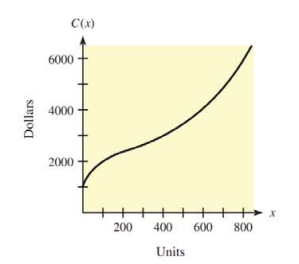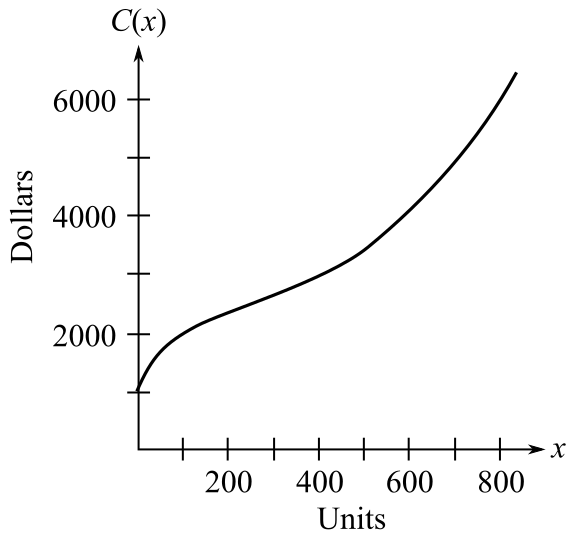Chapter 10.3, Problem 23E### Mathematical Applications for the ...

11th Edition
Ronald J. Harshbarger + 1 other
ISBN: 9781305108042

#### Solutions

Chapter
Section### Mathematical Applications for the ...

11th Edition
Ronald J. Harshbarger + 1 other
ISBN: 9781305108042
Textbook Problem

# The graphs in Problems 25 and 26 show total cost functions. For each problem(a) explain how to use the total cost graph to determine the level of production at which average cost is minimized.(b) determine that level of production.a)

To determine

The use of the total cost graph to determine the level of production at which average cost is minimized. The graph is,Explanation

Given information:

The provided graph is:

Explanation:

Consider the provided graph:

From the provided graph it is clear that:

If sketch a line between (0,0) to (x,

b)

To determine

The level of production from the provided graph.### Still sussing out bartleby?

Check out a sample textbook solution.

See a sample solution

#### The Solution to Your Study Problems

Bartleby provides explanations to thousands of textbook problems written by our experts, many with advanced degrees!

Get Started

#### Find more solutions based on key concepts